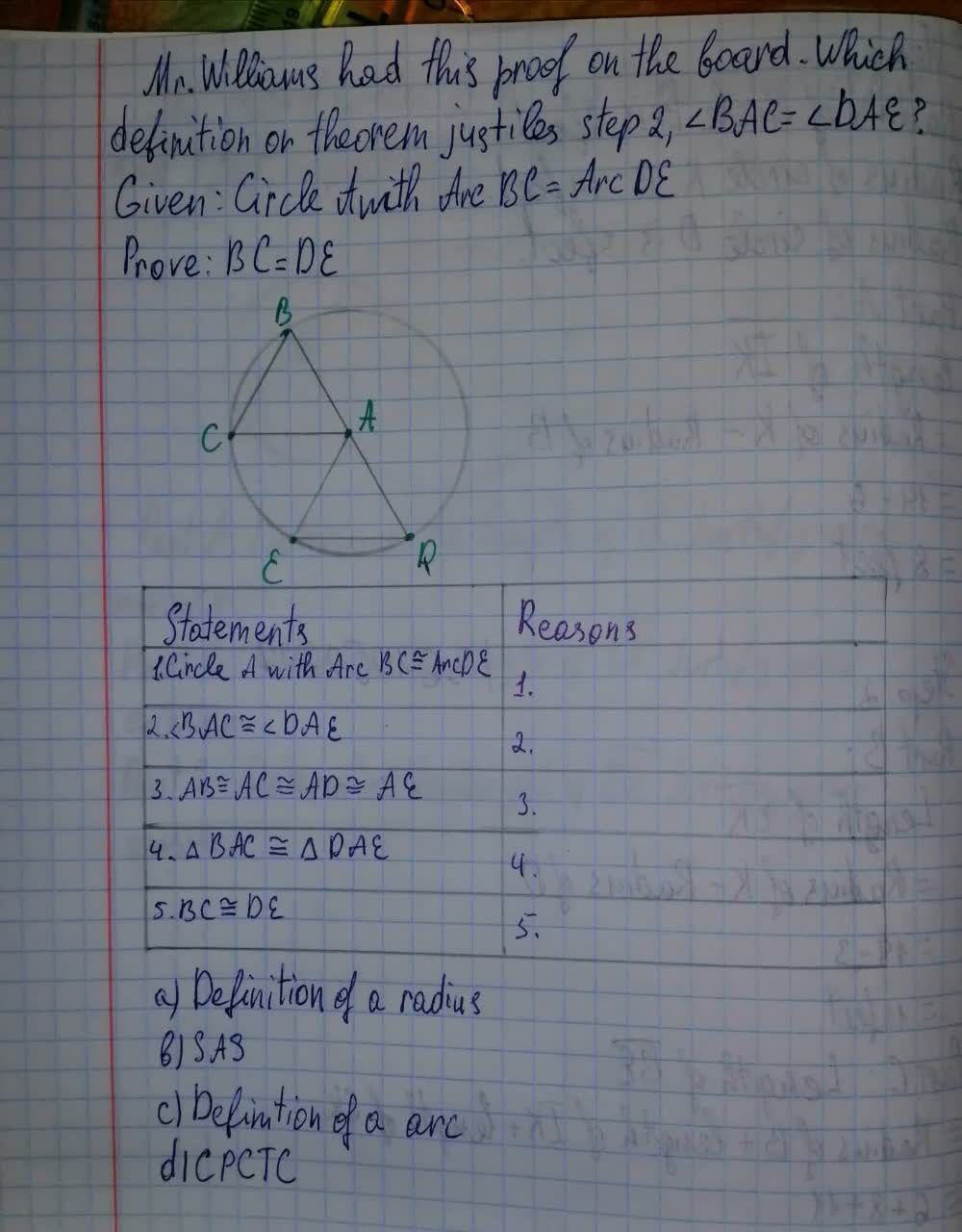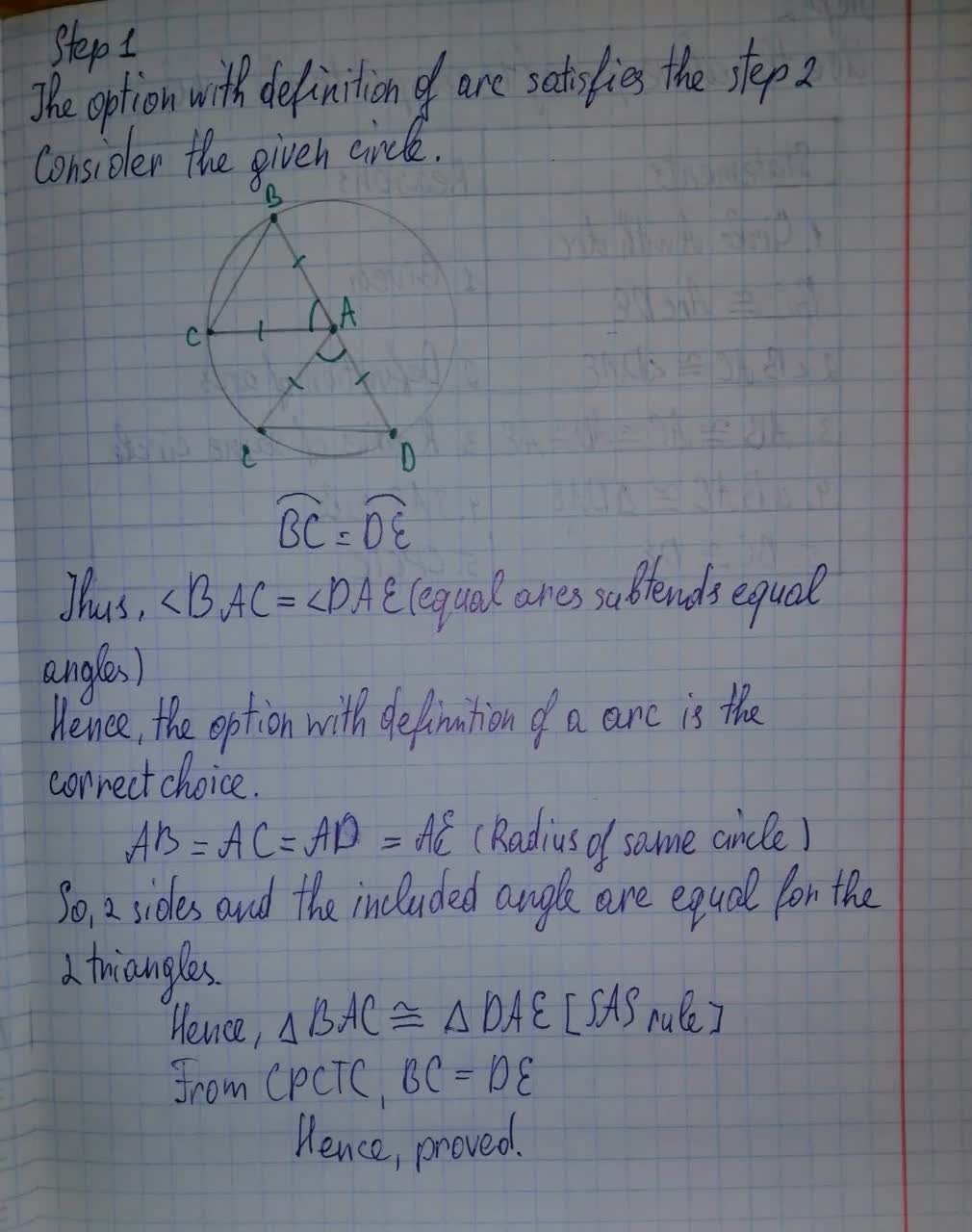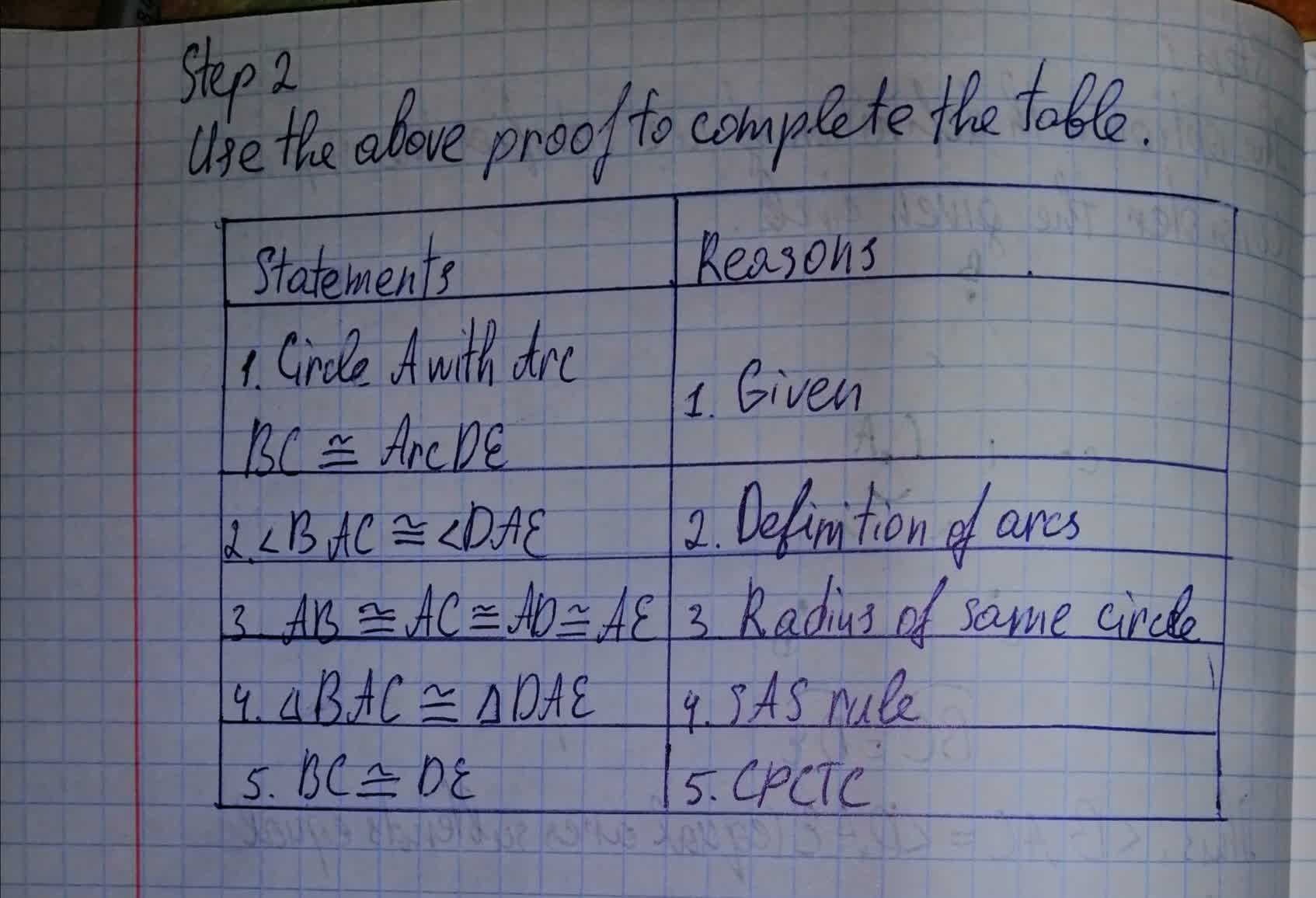Question# Which definition or theorem justfies step 2,\angle BAC = \angle DAE.Given: Circle A with Arc BC = Arc DE. Prove: BC=DE

Circles
ANSWEREDWhich definition or theorem justfies step 2,$$\displaystyle\angle{B}{A}{C}=\angle{D}{A}{E}$$
Given: Circle A with Arc BC = Arc DE
Prove: BC=DE